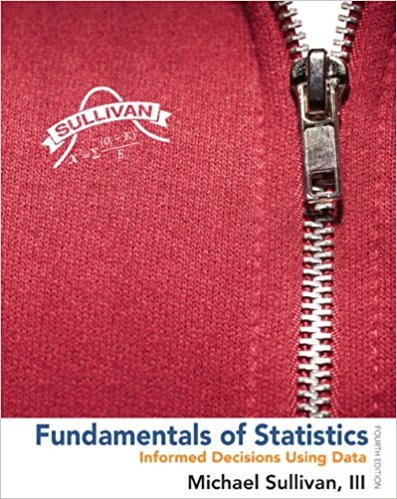×
×

# Solutions for Chapter Chapter 7.4: Fundamentals of Statistics 4th Edition## Full solutions for Fundamentals of Statistics | 4th Edition

ISBN: 9780321838704Solutions for Chapter Chapter 7.4

Solutions for Chapter Chapter 7.4
4 5 0 237 Reviews
27
4
##### ISBN: 9780321838704

Since 54 problems in chapter Chapter 7.4 have been answered, more than 310591 students have viewed full step-by-step solutions from this chapter. This expansive textbook survival guide covers the following chapters and their solutions. Chapter Chapter 7.4 includes 54 full step-by-step solutions. This textbook survival guide was created for the textbook: Fundamentals of Statistics, edition: 4. Fundamentals of Statistics was written by and is associated to the ISBN: 9780321838704.

Key Statistics Terms and definitions covered in this textbook
• 2 k p - factorial experiment

A fractional factorial experiment with k factors tested in a 2 ? p fraction with all factors tested at only two levels (settings) each

• `-error (or `-risk)

In hypothesis testing, an error incurred by rejecting a null hypothesis when it is actually true (also called a type I error).

A variation of the R 2 statistic that compensates for the number of parameters in a regression model. Essentially, the adjustment is a penalty for increasing the number of parameters in the model. Alias. In a fractional factorial experiment when certain factor effects cannot be estimated uniquely, they are said to be aliased.

• Analysis of variance (ANOVA)

A method of decomposing the total variability in a set of observations, as measured by the sum of the squares of these observations from their average, into component sums of squares that are associated with speciic deined sources of variation

• Arithmetic mean

The arithmetic mean of a set of numbers x1 , x2 ,…, xn is their sum divided by the number of observations, or ( / )1 1 n xi t n ? = . The arithmetic mean is usually denoted by x , and is often called the average

• Assignable cause

The portion of the variability in a set of observations that can be traced to speciic causes, such as operators, materials, or equipment. Also called a special cause.

• Axioms of probability

A set of rules that probabilities deined on a sample space must follow. See Probability

• Binomial random variable

A discrete random variable that equals the number of successes in a ixed number of Bernoulli trials.

• Causal variable

When y fx = ( ) and y is considered to be caused by x, x is sometimes called a causal variable

• Contingency table.

A tabular arrangement expressing the assignment of members of a data set according to two or more categories or classiication criteria

• Continuous uniform random variable

A continuous random variable with range of a inite interval and a constant probability density function.

• Convolution

A method to derive the probability density function of the sum of two independent random variables from an integral (or sum) of probability density (or mass) functions.

• Crossed factors

Another name for factors that are arranged in a factorial experiment.

• Dependent variable

The response variable in regression or a designed experiment.

• Discrete distribution

A probability distribution for a discrete random variable

• Error mean square

The error sum of squares divided by its number of degrees of freedom.

• Exhaustive

A property of a collection of events that indicates that their union equals the sample space.

• Experiment

A series of tests in which changes are made to the system under study

• F-test

Any test of signiicance involving the F distribution. The most common F-tests are (1) testing hypotheses about the variances or standard deviations of two independent normal distributions, (2) testing hypotheses about treatment means or variance components in the analysis of variance, and (3) testing signiicance of regression or tests on subsets of parameters in a regression model.

• Factorial experiment

A type of experimental design in which every level of one factor is tested in combination with every level of another factor. In general, in a factorial experiment, all possible combinations of factor levels are tested.

×